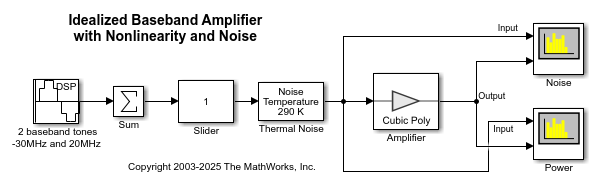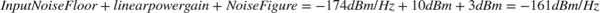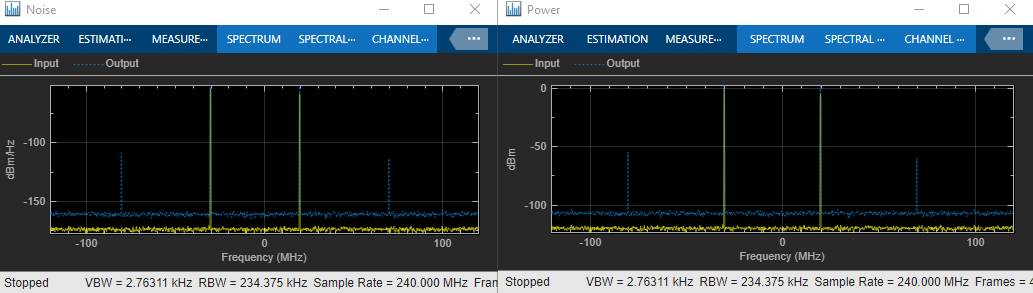# Idealized Baseband Amplifier with Nonlinearity and Noise

The example shows how to use the idealized baseband library Amplifier block to amplify a signal with nonlinearity and noise. The Amplifier uses the `Cubic Polynomial` model with a `Linear power gain` of 10 dB, an `Input IP3` nonlinearity of 30 dBm, and a `Noise figure` of 3 dB.### System Architecture

The DSP Sine Wave block inputs two complex baseband tones with a power level of -20 dBm and -25 dBm at frequencies of -30 MHz and 20 MHz. In this block you can also:

• Increase the samples per frame to increase the simulation speed.

• Use output complexity and phase offset to control the I-Q relationship of each baseband signal

• Control the bandwidth of the scopes using the inverse of the sample time parameter.

The Amplifier block only accepts a vector input. The Sum block combines the two baseband signals into a vector length equal to the samples per frame in the DSP Sine Wave block.

The Thermal Noise block creates a thermal noise floor input of -174 dBm/Hz.

### Simulation Analysis

The Amplifier block with `Linear power gain` of 10 dB outputs tone with magnitude -10 dBm and -15 dBm as seen in the Power plot. The Amplifier also increases the thermal noise floor to -161 dBm/Hz. You can calculate the output thermal noise using:The following plots illustrate the differences in the input and output noise floors. The spurs appear at 70 MHz (2*20 MHz + 30 MHz) and -80 MHz (2*(-30 MHz) - 20 MHz). This shows the third order intercept nature of the spurs.Increasing the Slider value from 1 to 10, shows nonlinear effects in the plots. These are the Noise and Power plots when the gain of the Slider is 10.